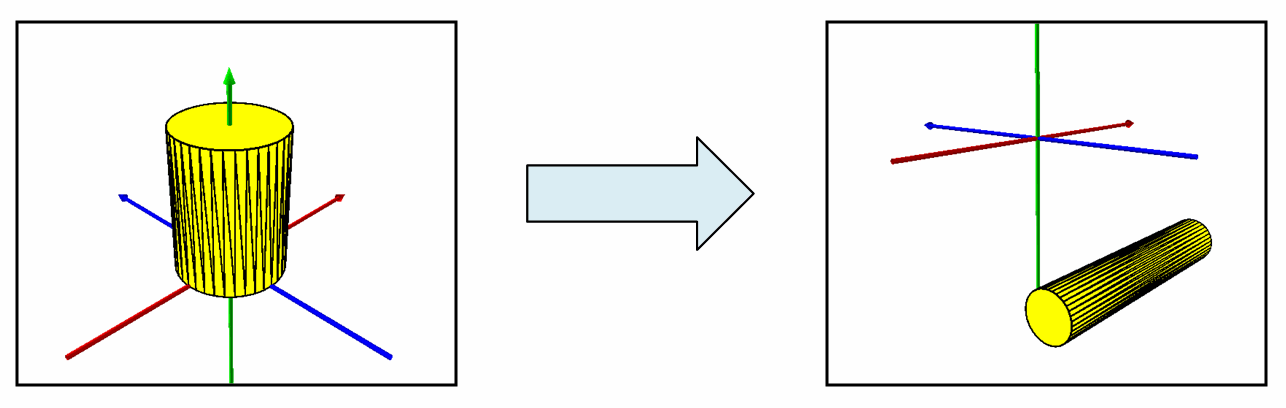ArcObjects Library Reference (Geometry)

# ITransform3D Interface

Provides access to methods for transforming 3D geometries using either specific parameters or arbitrary transformation objects.

#### Product Availability

Available with ArcGIS Engine, ArcGIS Desktop, and ArcGIS Server.

#### Members

DescriptionMove3D Moves the object by dx, dy and dz along the x, y, and z axes respectively.MoveVector3D Moves the object by an offset defined by a 3D vector.ProjectToPlane Generates a polygon footprint for the object in an arbitrary plane. The footprint may have multiple parts.RotateVector3D Rotates the object about axis defined by the specified vector through an angle measured in radians.Scale3D Scales the object about the specified origin point. sx, sy, and sz are the scaling factors for the x, y, and z dimensions repectively.Transform3D Applies an arbitrary 3D transformation.

#### CoClasses that implement ITransform3D

CoClasses and Classes Description
MultiPatch A collection of surface patches.
Multipoint An ordered collection of points; optionally has measure, height and ID attributes.
Path A sequence of connected segments.
Point A two dimensional point, optionally with measure, height, and ID attributes.
Polygon A collection of rings ordered by their containment relationship; optionally has measure, height and ID attributes.
Polyline An ordered collection of paths; optionally has measure, height and ID attributes.
Ring An area bounded by one, closed sequence of connected segments; optionally has measure, height and ID attributes at each vertex.
TriangleFan A continuous 3D fan of triangles, where each triangle after the first shares an edge with the preceding triangle, and all triangles share a common pivot point.
Triangles A collection of 3D triangles, where each consecutive triplet of vertices defines a new triangle
TriangleStrip A continuous 3D strip of triangles, where each triangle after the first shares an edge with the preceding triangle.

#### Remarks

These methods are intended to be called against top-level geometries only (Point, Polyline, Polygon, MultiPatch). To call a method against a Segment/Path or Ring, first add the part to a Polyline or Polygon container, respectively, and then call the appropriate method against the container.

[C#]public static void TransformMultiPatchGeometry()

{

const double XScale = 0.5;

const double YScale = 0.5;

const double ZScale = 2;

const double XOffset = -5;

const double YOffset = -5;

const double ZOffset = -8;

const double DegreesOfRotation = 90;

//Transform3D: Cylinder Scaled, Rotated, Repositioned Via Move3D(), Scale3D(), RotateVector3D()

IGeometry geometry = GetMultiPatchGeometry();

ITransform3D transform3D = geometry as ITransform3D;

//Stretch The Cylinder So It Looks Like A Tube

IPoint originPoint = ConstructPoint3D(0, 0, 0);

transform3D.Scale3D(originPoint, XScale, YScale, ZScale);

//Rotate The Cylinder So It Lies On Its Side

IVector3D axisOfRotationVector3D = ConstructVector3D(0, 10, 0);

double angleOfRotationInRadians = GetRadians(DegreesOfRotation);

transform3D.RotateVector3D(axisOfRotationVector3D, angleOfRotationInRadians);

//Reposition The Cylinder So It Is Located Underground

transform3D.Move3D(XOffset, YOffset, ZOffset);

}

private static IPoint ConstructPoint3D(double x, double y, double z)

{

IPoint point = ConstructPoint2D(x, y);

point.Z = z;

return point;

}

private static IPoint ConstructPoint2D(double x, double y)

{

IPoint point = new PointClass();

point.PutCoords(x, y);

return point;

}

private static double GetRadians(double decimalDegrees)

{

return decimalDegrees * (Math.PI / 180);

}

private static IVector3D ConstructVector3D(double xComponent, double yComponent, double zComponent)

{

IVector3D vector3D = new Vector3DClass();

vector3D.SetComponents(xComponent, yComponent, zComponent);

return vector3D;

}

#### .NET Samples

3D multipatch examples (Code Files: CompositeExamples DrawUtilities ExtrusionExamples Transform3DExamples)